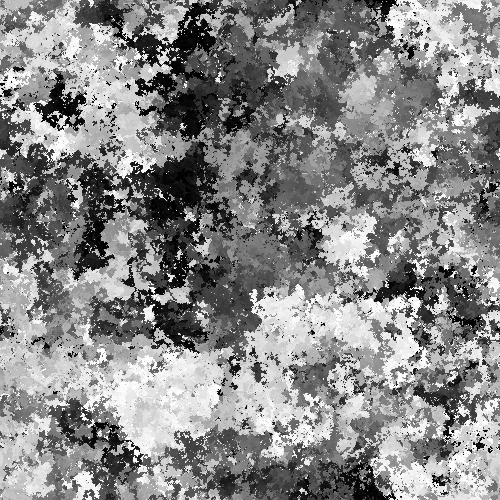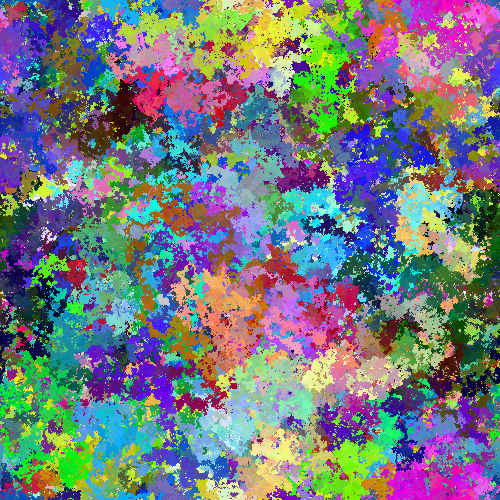# Random Walker

example processing animation random-walker generative-art

A random walker is a simple idea: start at some point, and then every frame, move in a random direction. As time goes on, you’ll randomly walk all around the screen. You can think of this like randomly scribbling on a piece of paper.

This might sound simple (and it is), but it’s also useful in all kinds of applications and explores the idea of emergence: the process of complicated (and sometimes beautiful) patterns emerging from simple rules.

I could babble all day about emergence (the random walker is one of my favorite algorithms), so let’s just get to the code:

``````float x;
float y;

void setup() {
size(200, 100);

//start in middle of screen
x = width/2;
y = height/2;

//gray background
background(200);

//make the simulation faster
frameRate(1000);
}

void draw() {

stroke(0);

//randomly move
x += random(-1, 1);
y += random(-1, 1);

//prevent going off left or right
if(x < 0){
x = width;
}
if(x > width){
x = 0;
}

//prevent going off top or bottom
if(y < 0){
y = height;
}
if(y > height){
y = 0;
}

//draw the point
point(x, y);
}
``````

This code does what we described above: starts a point in the middle of the screen, randomly moves that point every frame, and then just draws the point.Code Editor

See the Pen by Happy Coding (@KevinWorkman) on CodePen.

From here we could expand our code to include another random walker:

``````float blackX;
float blackY;

float whiteX;
float whiteY;

void setup() {
size(200, 100);

blackX = width*.25;
blackY = height/2;

whiteX = width*.75;
whiteY = height/2;

background(128);

frameRate(1000);
}

void draw() {

stroke(0);

blackX += random(-1, 1);
blackY += random(-1, 1);

if(blackX < 0){
blackX = width;
}
if(blackX > width){
blackX = 0;
}

if(blackY < 0){
blackY = height;
}
if(blackY > height){
blackY = 0;
}

point(blackX, blackY);

stroke(255);

whiteX += random(-1, 1);
whiteY += random(-1, 1);

if(whiteX < 0){
whiteX = width;
}
if(whiteX > width){
whiteX = 0;
}

if(whiteY < 0){
whiteY = height;
}
if(whiteY > height){
whiteY = 0;
}

point(whiteX, whiteY);

}
``````

This program adds another set of variables and does the exact same logic.Code Editor

See the Pen by Happy Coding (@KevinWorkman) on CodePen.

Or we could go back to a single walker, but add `random(-1, 1)` to the value we pass into the `stroke()` function. In other words, we could walk the color as well as the position.Or we could do the same thing to the red, green, and blue parameters:## Tweak Ideas

• Base the random movement off of a heading (an angle) that you randomly change. This will result in smoother movement.
• Add more random walkers: maybe one for each color of the rainbow, or maybe different walkers with different speeds.